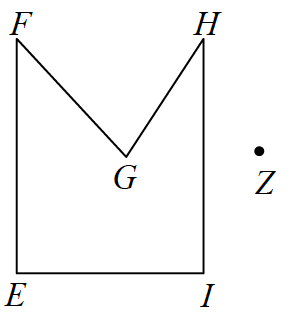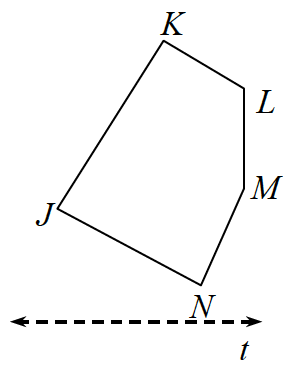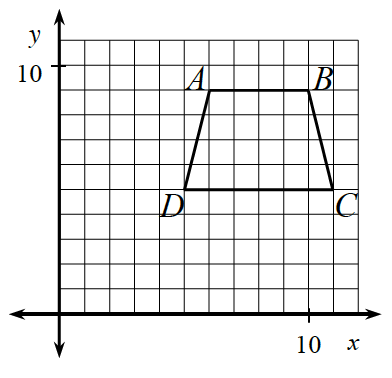### Home > INT1 > Chapter 4 > Lesson 4.1.4 > Problem4-38

4-38.

Trace the figures in parts (a) and (b) onto your paper and perform the indicated transformations. Copy the figure from part (c) onto graph paper and perform the indicated transformation. Label each image with prime notation $(A \rightarrow A^\prime)$.

1. Rotate $EFGHI$ $90º$ counter-clockwise $(\circlearrowleft)$ about point $Z$Review the Math Notes box in Lesson 3.1.4.

1. Reflect $JKLMN$ over line $t$Review the Math Notes box in Lesson 3.1.4.

1. Translate $ABCD$ down $3$ units and left $4$ unitsRecall a translation moves every point a specified amount and direction.

Use the eTool below to solve each part.
Click the link at right for the full version of the eTool: 4-38 HW eTool